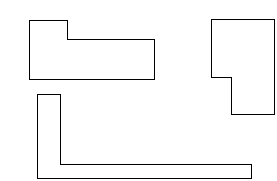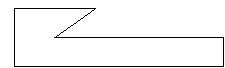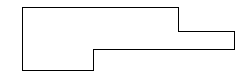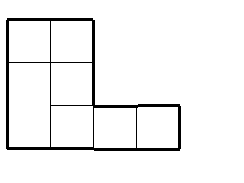Assessment Teacher Directed

Perimeter and Area

Hexaright Problems

A new kind of shape was made and named hexaright.

Here is a definition: A hexaright is a hexagon in which each pair of adjacent sides is perpendicular.

Here are some examples of hexarights:This is not a hexaright because not all pairs of adjacent sides are perpendicular.1. This is not a hexaright either. Why not?2. This hexaright has been drawn on some centimeter graph paper. Find the perimeter and the area of this hexaright:Perimeter: __________________

Area: __________________

3. This hexaright has also been drawn on some centimeter graph paper. Find the perimeter and area of this hexaright:Perimeter: __________________

Area: ___________________

4. Here's a hexaright with a perimeter of 24 cm.

picture missing:

What is the area of this hexaright? _________

5. There are lots of different hexarights with a perimeter of 24 cm. On a separate piece of paper, draw two different hexarights, each one with a perimeter of 24 cm.

6. Draw one more hexaright with perimeter 24 cm, and make the area as large as you can. You can draw it in the space below or on another piece of paper.

What is the area of the hexaright you just drew? __________

7. What did you find about the areas of hexarights with a perimeter of 24 cm?

(1993). Measuring UP: Prototypes for Mathematics Assessment.. Mathematical Sciences Education Board National Research Council. Washington, DC: National Academy Press.